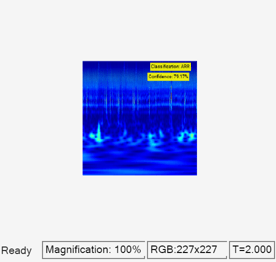# Classify ECG Signals in Simulink Using Deep Learning

This example shows how to use wavelet transforms and a deep learning network within a Simulink (R) model to classify ECG signals. This example uses the pretrained convolutional neural network from the Classify Time Series Using Wavelet Analysis and Deep Learning example of the Wavelet Toolbox™ to classify ECG signals based on images from the CWT of the time series data. For information on training, see Classify Time Series Using Wavelet Analysis and Deep Learning (Wavelet Toolbox).

### ECG Data Description

This example uses ECG data from PhysioNet database. It contains data from three groups of people:

1. Persons with cardiac arrhythmia (ARR)

2. Persons with congestive heart failure (CHF)

3. Persons with normal sinus rhythms (NSR)

It includes 96 recordings from persons with ARR, 30 recordings from persons with CHF, and 36 recordings from persons with NSR. The `ecg_signals` MAT-file contains the test ECG data in time series format. The image classifier in this example distinguishes between ARR, CHF, and NSR.

### Algorithmic Workflow

The block diagram for the algorithmic workflow of the Simulink model is shown.### ECG Deep Learning Simulink Model

The Simulink model for classifying the ECG signals is shown. When the model runs, the `Video Viewer` block displays the classified ECG signal.

```open_system('ecg_dl_cwtMDL'); ```### ECG Preprocessing Subsystem

The `ECG Preprocessing` subsystem contains a `MATLAB Function` block that performs CWT to obtain scalogram of the ECG signal and then processes the scalogram to obtain an image. It also contains an `Image Classifier` block from the Deep Learning Toolbox™ that loads the pretrained network from `trainedNet.mat` and performs prediction for image classification based on SqueezeNet deep learning CNN.

```open_system('ecg_dl_cwtMDL/ECG Preprocessing'); ```The `ScalogramFromECG` function block defines a function called `ecg_to_scalogram` that:

• Uses 65536 samples of double-precision ECG data as input.

• Create time frequency representation from the ECG data by applying Wavelet transform.

• Obtain scalogram from the wavelet coefficients.

• Convert the scalogram to image of size (227-by-227-by-3).

The function signature of `ecg_to_scalogram` is shown.

```type ecg_to_scalogram ```
```function ecg_image = ecg_to_scalogram(ecg_signal) % Copyright 2020 The MathWorks, Inc. persistent jetdata; if(isempty(jetdata)) jetdata = colourmap(128,'single'); end % Obtain wavelet coefficients from ECG signal cfs = cwt_ecg(ecg_signal); % Obtain scalogram from wavelet coefficients image = ind2rgb(im2uint8(rescale(cfs)),jetdata); ecg_image = im2uint8(imresize(image,[227,227])); end ```

### ECG Postprocessing

The `ECG Postprocessing` MATLAB function block defines the `label_prob_image` function that finds the label for the scalogram image based on the highest score from the scores outputed by the image classifier. It outputs the scalogram image with the label and confidence overlayed.

```type label_prob_image ```
```function final_image = label_prob_image(ecg_image, scores, labels) % Copyright 2020 The MathWorks, Inc. scores = double(scores); % Obtain maximum confidence [prob,index] = max(scores); confidence = prob*100; % Obtain label corresponding to maximum confidence label = erase(char(labels(index)),'_label'); text = cell(2,1); text{1} = ['Classification: ' label]; text{2} = ['Confidence: ' sprintf('%0.2f',confidence) '%']; position = [135 20 0 0; 130 40 0 0]; final_image = insertObjectAnnotation(ecg_image,'rectangle',position,text,'TextBoxOpacity',0.9,'FontSize',9); end ```

### Run the Simulation

To verify the algorithm and display the labels and confidence score of the test ECG signal loaded in the workspace, run the simulation.

```set_param('ecg_dl_cwtMDL', 'SimulationMode', 'Normal'); sim('ecg_dl_cwtMDL'); ```### Code Generation

With GPU Coder™, you can accelerate the execution of model on NVIDIA® GPUs and generate CUDA® code for model. See the Code Generation for a Deep Learning Simulink Model to Classify ECG Signals (GPU Coder) for more details.

### Cleanup

```close_system('ecg_dl_cwtMDL/ECG Preprocessing'); close_system('ecg_dl_cwtMDL'); ```